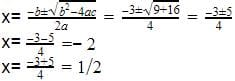Which of the given is the set of zeroes of th...

### Related TestWhich of the given is the set of zeroes of the polynomial p(x) = 2x3 + x2 – 5x + 2
• a)
1/2 , -1 , -2
• b)
1/2 , 1 , -2
• c)
-1/2 , -1 , -2
• d)
-1/2 , 1 , -2Aadhar AcademyWe have the polynomial 2x3+x2-5x+2
By Hit and trial method, x=1 is the solution.
Dividing the polynomial by x-1
We get quotient as = 2x2+3x-21 Crore+ students have signed up on EduRev. Have you?Peter Parker
Which of the given is the set of zeroes of the polynomial p(x) = 2x3+ x2– 5x + 2a)1/2 , -1 , -2b)1/2 , 1 , -2c)-1/2 , -1 , -2d)-1/2 , 1 , -2Correct answer is option 'B'. Can you explain this answer?
Option B is correct...View courses related to this question Explore Class 10 courses
 Explore Class 10 coursesView courses related to this questionAbhishek Kumar
B

• ### Let alpha and beta are the zeroes of quadratic polynomial 2x^2-5x-6 then fo... more(Scan QR code)